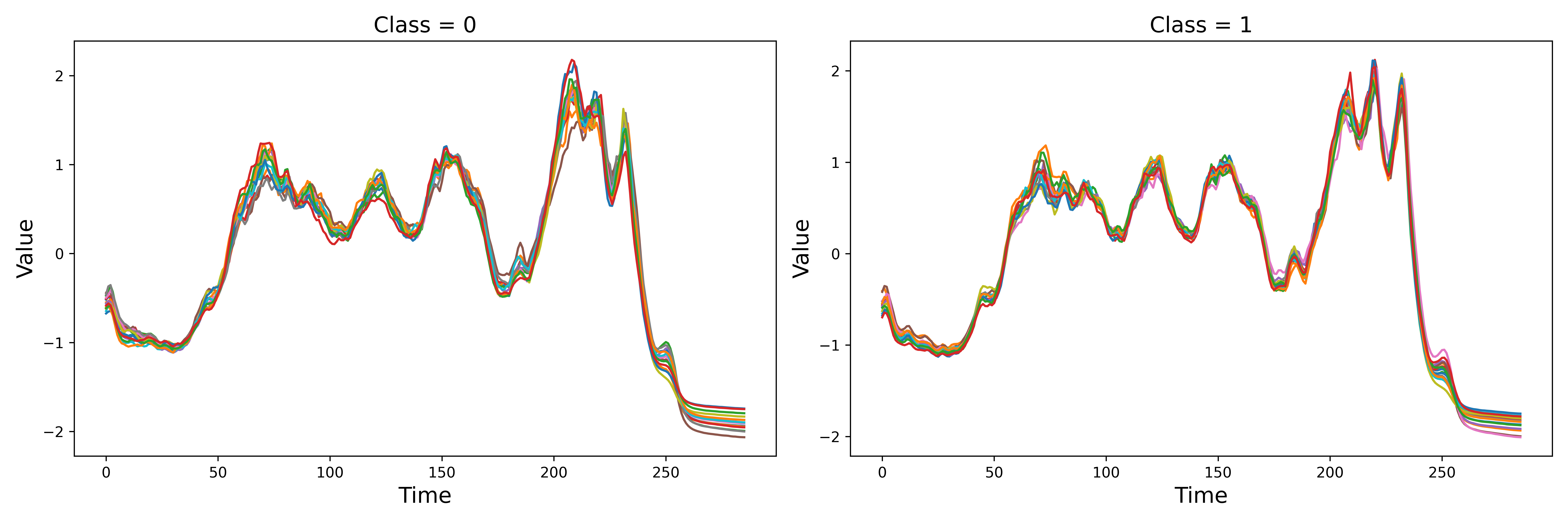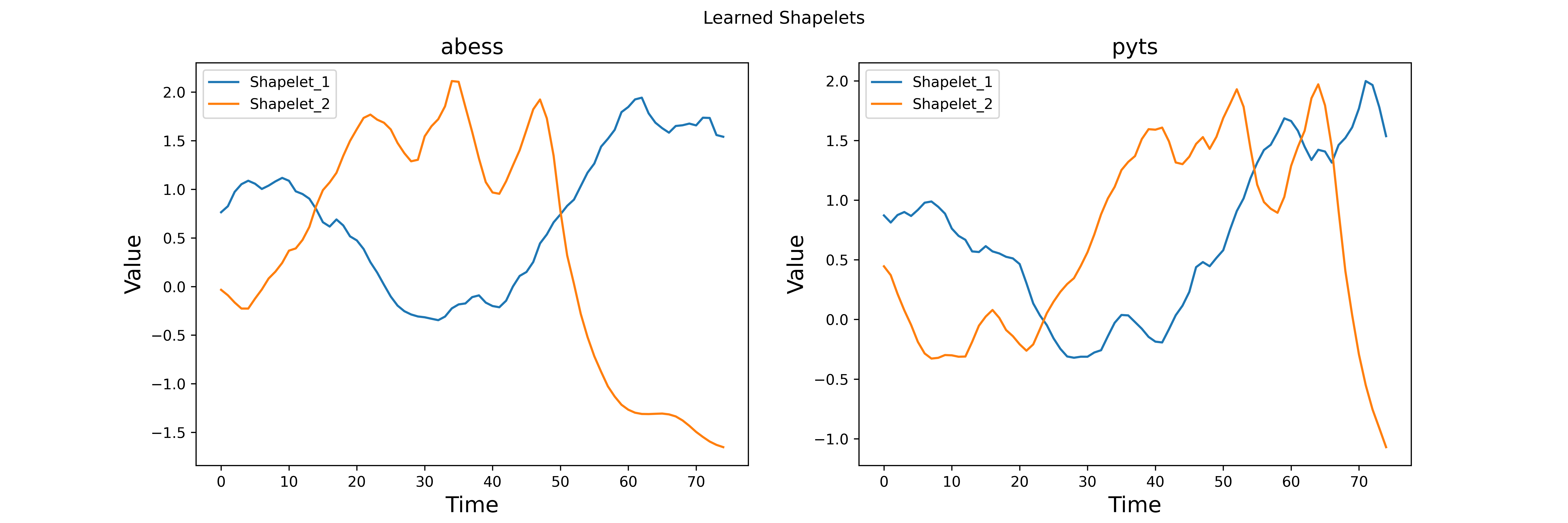# Work with pyts¶

`pyts` is a Python package dedicated to time series classification. It aims to make time series classification easily accessible by providing preprocessing and utility tools, and implementations of several time series classification algorithms. In this example, we will mainly focus on the shapelets-based algorithms.

Shapelets learning is a new primitive in time series classification. Shapelets are defined as subsequences of time series that are in some sense maximally representative of a class. Informally, in a binary classification task, a shapelet is discriminant if it is present in most series of one class and absent from series of the other class. `ShapeletTransform` is a powerful method implemented by `pyts` to perform shapelets-based feature transformation. Actually, `abess` also works well with shapelets-based methods. This example shows how to effectively select discriminant shapelets with `abess`.

```import numpy as np
import matplotlib.pyplot as plt
import time
import warnings
warnings.filterwarnings('ignore')
from abess.linear import LogisticRegression
from sklearn.svm import LinearSVC
from sklearn.pipeline import make_pipeline
from pyts.transformation import ShapeletTransform
```

## Data¶

In this example, we use the buint-in coffee dataset in `pyts` to perform shapelets learning. It has two classes, 0 and 1. So, this is a binary classification task. Both train dataset and test dataset have 28 time series and the dimension of each time series is 286. We plot the time series in the train dataset.

```X_train, X_test, y_train, y_test = load_coffee(return_X_y=True)
print("X_train shape: ", X_train.shape)
print("X_test shape: ", X_test.shape)

fig, (ax1, ax2) = plt.subplots(1, 2, figsize=(15, 5), dpi=600)
ax1.plot(X_train[y_train == 0].T)
ax1.set_title("Class = 0", fontsize=15)
ax1.set_xlabel("Time", fontsize=15)
ax1.set_ylabel("Value", fontsize=15)
ax2.plot(X_train[y_train == 1].T)
ax2.set_title("Class = 1", fontsize=15)
ax2.set_xlabel("Time", fontsize=15)
ax2.set_ylabel("Value", fontsize=15)
fig.tight_layout()
plt.show()
``````X_train shape:  (28, 286)
X_test shape:  (28, 286)
```

## Learning shapelets with `abess`¶

To select discriminant shapelets, we first collect all subsequences with predefined length and step as the candidates. Then we transform the original time series by computing the distance between them to each subsequence. Therefore, the original time series are transformed to some ultra high dimensional vectors. Finally, we perform binary classification and shapelets selection simultaneously with `LogisticRegression` implemented by `abess`.

```class abessShapelet(object):

def __init__(self, X_train, X_test, y_train, y_test, len_shapelet=None, step=None):
self.X_train = X_train
self.X_test = X_test
self.y_train = y_train
self.y_test = y_test
self.n, self.p = X_train.shape
if len_shapelet == None:
len_shapelet = int(self.p / 4)
if step == None:
step = int(len_shapelet / 2)
num_each = 1 + (self.p - len_shapelet) // step
self.shapelets = []
for i in range(self.n):
for j in range(num_each):
col = j*step
self.shapelets.append(self.X_train[i, col:(col+len_shapelet)])
self.shapelets = np.array(self.shapelets)

def distant(self, x, y):
assert x.ndim == 1 and y.ndim == 1
n_x = len(x)
n_y = len(y)
if n_x <= n_y:
dist = np.zeros(n_y-n_x+1)
for i in range(n_y-n_x+1):
shapelet = y[i:i+n_x]
dist[i] = np.sum((x-shapelet)**2)
return dist.min()
else:
dist = np.zeros(n_x-n_y+1)
for i in range(n_x-n_y+1):
shapelet = x[i:i+n_y]
dist[i] = np.sum((y-shapelet)**2)
return dist.min()

def featureTransform(self, X, shapelets, index=None):
if index is None:
index = np.arange(shapelets.shape)
n, p = X.shape
num_shapelets, k = shapelets.shape
new_feature = np.zeros((n, num_shapelets))
for i in range(n):
for j in index:
new_feature[i, j] = self.distant(X[i], shapelets[j])
return new_feature

def fit_predict(self, size=None):
X_train_new = self.featureTransform(self.X_train, self.shapelets)
model = LogisticRegression(support_size=size)
model.fit(X_train_new, self.y_train)
self.index = np.nonzero(model.coef_)
X_test_new = self.featureTransform(
self.X_test, self.shapelets, self.index)
y_pred = model.predict(X_test_new)
return y_pred
```

In the following, we perform shapelets learning using `abessShapelet`. We print the performance and execution time.

```t1 = time.time()
aShapelet = abessShapelet(X_train, X_test, y_train, y_test, len_shapelet=75)
y_pred = aShapelet.fit_predict(size=2)
score_abess = (y_pred == y_test).mean()
t2 = time.time()
print("score_abess: ", round(score_abess, 2))
print("time_abess : {}s".format(round(t2 - t1, 2)))
```
```score_abess:  0.96
time_abess : 6.98s
```

## Learning shapelets with `pyts`¶

We compare our method with the one implemented in `pyts`, which is a two-step procedure. First, it selects discriminant shapelets based on mutual information. Then, a support vector machine is applied to perform binary classification with transformed time series based on those selected shapelets. Analogously, we print the performance and execution time.

```t3 = time.time()
shapelet = ShapeletTransform(n_shapelets=2, window_sizes=, sort=True)
svc = LinearSVC()
clf = make_pipeline(shapelet, svc)
clf.fit(X_train, y_train)
score_pyts = clf.score(X_test, y_test)
t4 = time.time()
print("score_pyts: ", round(score_pyts, 2))
print("time_pyts : {}s".format(round(t4 - t3, 2)))
```
```score_pyts:  0.96
time_pyts : 28.11s
```

It can be seen from the above results that the linear classifier `abessShapelet` obtains the same performance with the method implemented by `pyts` while the running time is much shorter.

## Plot: learned shapelets¶

The following figure shows the discriminant shapelets selected by these two methods.

```fig, (ax1, ax2) = plt.subplots(1, 2, figsize=(15, 5), dpi=600)

ax1.plot(aShapelet.shapelets[aShapelet.index], label="Shapelet_1")
ax1.plot(aShapelet.shapelets[aShapelet.index], label="Shapelet_2")
ax1.legend()
ax1.set_title("abess", fontsize=15)
ax1.set_xlabel("Time", fontsize=15)
ax1.set_ylabel("Value", fontsize=15)

ax2.plot(shapelet.shapelets_, label="Shapelet_1")
ax2.plot(shapelet.shapelets_, label="Shapelet_2")
ax2.legend()
ax2.set_title("pyts", fontsize=15)
ax2.set_xlabel("Time", fontsize=15)
ax2.set_ylabel("Value", fontsize=15)

plt.suptitle("Learned Shapelets")
plt.show()
```sphinx_gallery_thumbnail_path = 'Tutorial/figure/pyts.png'

Total running time of the script: ( 0 minutes 39.486 seconds)

Gallery generated by Sphinx-Gallery